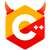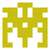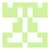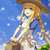# 3017. 计算n!右端0的个数(I)$$f(n）= \begin{cases} 0 & n < 5 \ f(n-1) + g(n) & n \ge 5 \end{cases}$$

#include <iostream>

int g(int n) {
int ret = 0;
while (n % 5 == 0) {
n /= 5;
++ret;
}
return ret;
}

int f(int n) {
if (n < 5)
return 0;
else
return f(n - 1) + g(n);
}

int main() {
int t;
scanf("%d", &t);
for (int i = 0; i < t; ++i) {
int n;
scanf("%d", &n);
printf("case #%d:\n%d\n", i, f(n));
}
}


f(0) = 0        f(1) = 0        f(2) = 0
f(3) = 0        f(4) = 0        f(5) = 1
f(6) = 1        f(7) = 1        f(8) = 1
f(9) = 1        f(10) = 2       f(11) = 2
f(12) = 2       f(13) = 2       f(14) = 3
f(15) = 3       f(16) = 3       f(17) = 3
f(18) = 3       f(19) = 3       f(20) = 4
f(21) = 4       f(22) = 4       f(23) = 4
f(24) = 4       f(25) = 6       f(26) = 6
f(27) = 6       f(28) = 6       f(29) = 7
f(30) = 7       f(31) = 7       f(32) = 7
f(33) = 7       f(34) = 7       f(35) = 8
f(36) = 8       f(37) = 8       f(38) = 8
f(39) = 8       f(40) = 9       f(41) = 9
f(42) = 9       f(43) = 9       f(44) = 10
f(45) = 10      f(46) = 10      f(47) = 10
f(48) = 10      f(49) = 10      f(50) = 12


fn g(mut x: u32) -> u32 {
let mut ret = 0;
while x % 5 == 0 {
x /= 5;
ret += 1;
}
ret
}

fn f(n: u32) -> u32 {
if n < 5 {
0
} else {
f(n - 1) + g(n)
}
}### include

using namespace std;

int main()
{
int n;
cin >> n;
int i;
for(i = 0; i < n; i++){
int num;
int cnt;
cin >> num;
if(num >= 1 && num < 5){
cnt = 0;
}
if(num >= 5 && num < 10){
cnt = 1;
}
if(num >= 10 && num < 15){
cnt = 2;
}
if(num >= 15 && num < 20){
cnt = 3;
}
if(num == 20){
cnt = 4;
}
cout << “case #” << i << “:” << endl;
cout << cnt << endl;
}
return 0;
}### include

void FactorialZeros(int n){
int b;
b=n/5;
printf(“%d\n”,b);
}
int main()
{
int N,n;
scanf(“%d”,&N);
int i;
int a[N];
for(i=0;i<N;i++){
scanf(“%d”,&a[i]);}
for(i=0;i<N;i++){
printf(“case #%d:\n”,i);
FactorialZeros(a[i]);}
}#include <cstdio>

inline unsigned get_uint() {
unsigned ans = 0;
char ch = getchar();
while (ch < '0' || '9' < ch)
ch = getchar();
while ('0' <= ch && ch <= '9') {
ans = ans * 10 + ch - '0';
ch = getchar();
}
return ans;
}

int main() {
unsigned n = get_uint();
for (int i = 0; i < n; ++i)
printf("case #%i:\n%i\n", i, get_uint() / 5);
}